Back

# Goldilocks and the Three Bears: ODTK Edition

Orbit Determination Tool Kit (ODTK) provides orbit determination and orbit analysis support for the entire life cycle of satellites and their tracking systems. In its simplest terms, ODTK allows you to process a wide variety of traditional and non-traditional measurements through a sequential Kalman filter with custom process noise and then produce various plots and charts to analyze and confirm your results. But what results should you care about?

The graph that almost everyone starts with is residuals or residual ratios. Residuals are the difference between what you observed and what the force models computed. Residuals are used to check how dispersed the tracking measurements are with respect to the estimated solution. Residual ratios are the ratio between the residual and its variance, and it is useful because it becomes a unit-less quantity. Ideally, residual ratios will all fall between ±3σ boundaries and your residual ratio plot might look like this: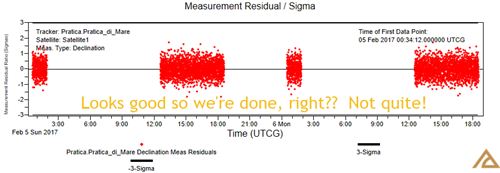The residual ratios are falling nicely between the ±3σ bounds but they are not taking up the whole span, which is a sign that the white noise sigma values on the measurements are too large and that the residuals do not have a Normal distribution. The white noise is the random variation about the measured mean; the white noise sigma is a parameter in the measurement statistics that is meant to represent the uncertainty in the measurements due to thermal noise. Now we enter the Goldilocks story where we are trying to find the white noise sigma on our declination measurements that is just the right size. There are two very useful plots in ODTK that can help us with this. One is a histogram and the other is called a QQ plot.

The histogram is used to verify if the observations have a normal distribution. The normal density is shown as a red line and several blue bars display the actual percentage distribution of the samples. The ideal condition would be to have a distribution that strictly follows the Gaussian one. It is important to note that the Histogram is only useful when displaying a single data type from a single tracking sensor. The histogram for the above residual ratios is: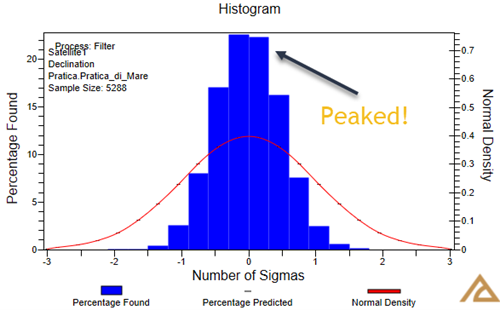A peaked histogram indicates that the white noise sigma is too large. Sometimes it can be hard to tell if a histogram has a Normal distribution when there are not enough samples (i.e., sparse data). In cases where there is sparse data, the Residual Ratio QQ plot really shines. A QQ plot ("Q" stands for "Quantile") plots the residual ratios with Royston acceptance boundaries. The filter residual ratio quantile points are plotted against the same test quantile from a Normal distribution. An ideal QQ plot would have the filter results matching the Normal distribution, creating a line with a slope of one. Note that the QQ plot, like a histogram, is only useful when displaying a single data type from a single tracking sensor. For this same filter run, we see the following QQ plot: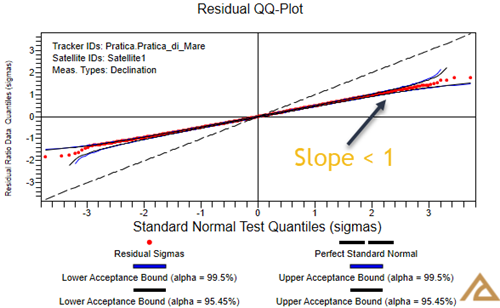The slope is less than one, which is another indicator that the white noise sigma is too large. We’ve looked at the case for a too large of a white noise sigma. It’s time for Goldilocks to try a smaller white noise sigma.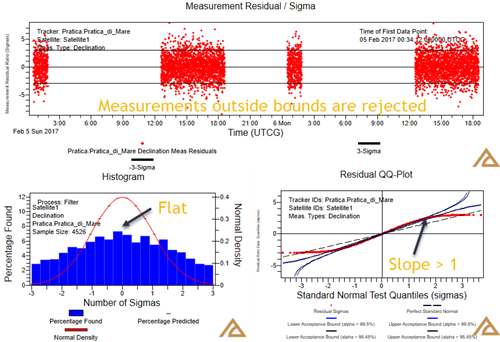This case above is more of a red-flag than the too-large case since measurements are now falling outside of the ±3σ bounds and are, therefore, rejected. This situation corresponds to a flattened histogram and a QQ plot with a slope greater than one. Now if we go somewhere in the middle and find the perfect white noise sigma, below is what we should see in ODTK: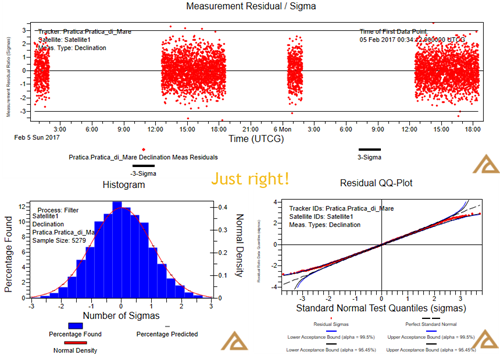### To sum this all up Goldilocks Style:

White Noise Sigma Too Big White Noise Sigma Too Small White Noise Sigma Just Right!
Residual ratios are bunched within the editing threshold Residual ratios are consistently outside the editing threshold Residual ratios take up the entire span of the editing threshold
Histogram is peaked Histogram is squashed Histogram approaches normal distribution
QQ plot has slope less than 1 QQ plot has slope greater than 1 QQ plot has slope close to 1